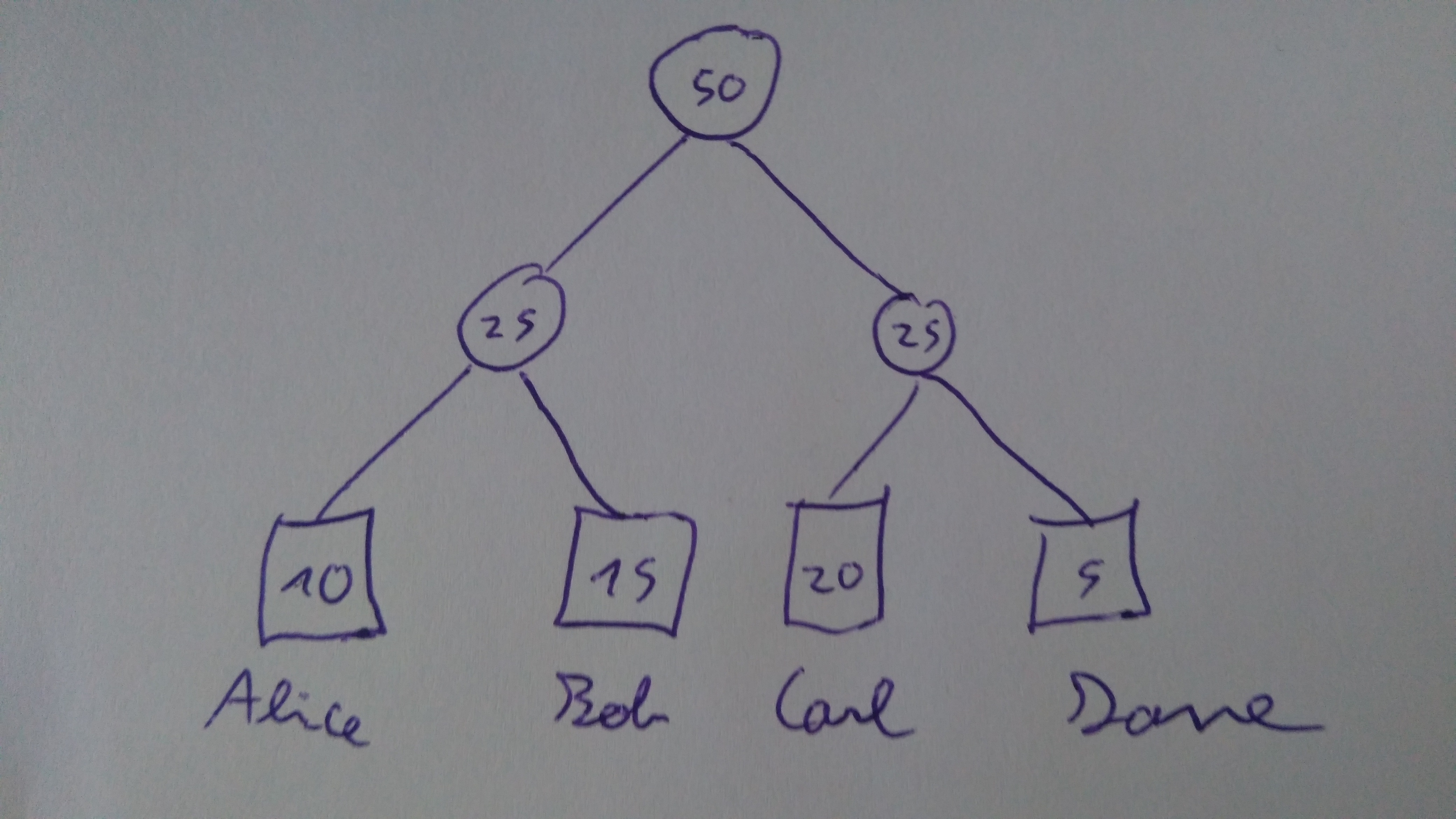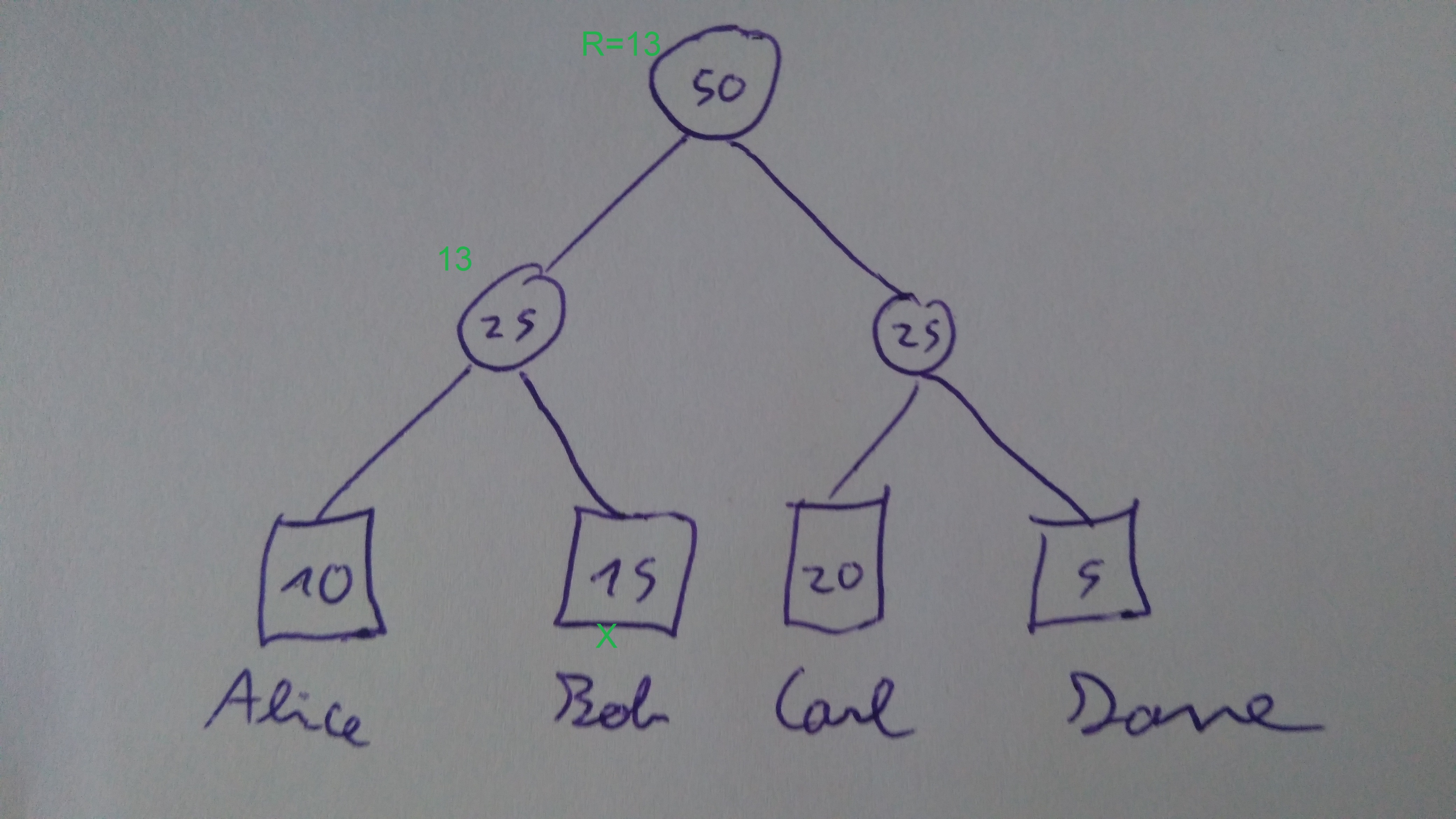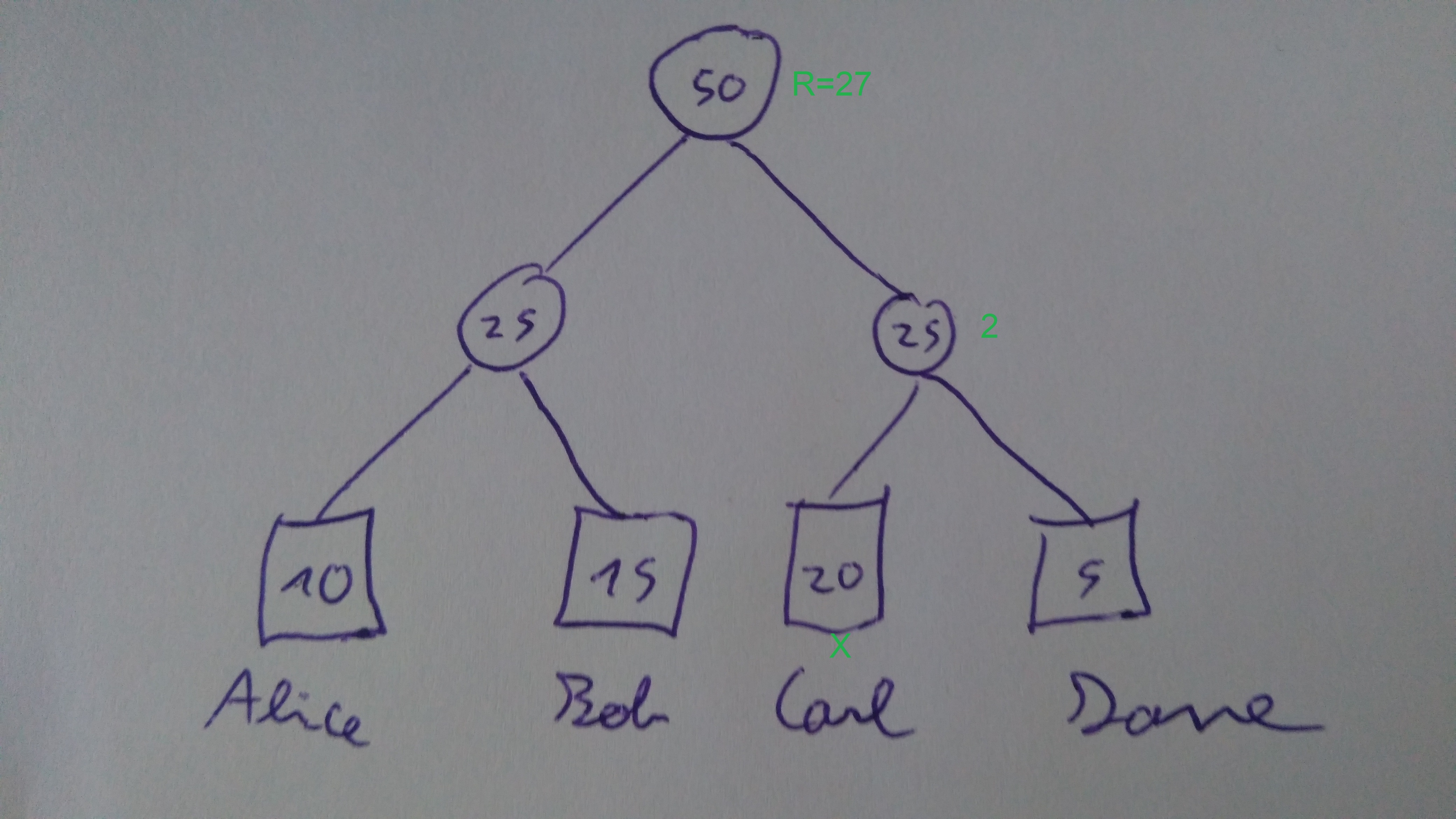Skip to content

# kleros/kleros

Have a question about this project? Sign up for a free GitHub account to open an issue and contact its maintainers and the community.

By clicking “Sign up for GitHub”, you agree to our terms of service and privacy statement. We’ll occasionally send you account related emails.

Already on GitHub? Sign in to your account

# [Bug Bounty: 50 ETH] Sortition Sum Tree #115

Closed
opened this issue Feb 5, 2019 · 2 comments

Projects
None yet
Member

# Sortition Sum Tree Bounties

This bug bounty is posted on solidified.
You can report bugs on solidified or by sending a mail to clement@kleros.io. Bugs are rewarded up to 50 ETH according to the classification indicated on solidified.

## Sortition Sum Tree

### Introductory example

In Kleros liquid, instead of using segments, we would use a sortition sum tree. This would allow depositing and taking back PNK in almost real time.
With
Alice: 10
Bob: 15
Carl: 20
Dave: 5

We would have the following sum-tree:The root tells us that we should draw r in [0,50[.
If we have r=13, we see it's lower than the left value of 25, so continue the search into it.
It's also higher than the left value 10, so we end up in the Bob leaf.Now, if we draw r=27.
It's higher than the left node, so we continue into the right one, but subtract the value of the left on. So we continue the search with r=27-25=2. This is lower than the left leaf, so we end up into Carl leaf.We can find out which node is drawn in logarithmic time (assuming the tree is balanced).

### k-ary

In order to save on write operations, we can use a K-ary tree (tree where nodes can have up to `K` childs).
Assuming the tree to be balanced, finding the right user would be in `O(K log(n)/log(K))` and the amount of write operations would decrease to `O(log(n)/log(K))`. This way, we can save a substantial amount of write operations at the price of some read and memory ones.
We can set up `K` to be optimal for an assumed amount of jurors (for example 10 000 jurors).

### Keeping the tree pseudo-balanced

To keep it pseudo-balanced when nodes are added, instead of saving the amount of descendants of each node (which would take additional space), we can:

• When a node is removed:
-- Deactivate it (by setting it to `0`) and add its number to a stack (which can be simply represented as a list).
-- Update all ascendants by subtracting `v`, where `v` is the value the node had before.

• When a node is added:
-- If the stack is empty:
---- Add the node `i=n` where `n` is the current number of nodes. If this node descend from a leaf (i.e it's the first child of a node which can be checked by `(i-1)%K == 0`), also copy the leaf value to a new node i'=i+1 and turn the leaf to a sum-node (with identical value).
-- Else:
---- Pop up the node on the top of the stack, reactivate it and use it.
-- Update all the ascendant nodes by adding `v` where `v` is the value which was added.

• When a node is updated:
---- Update all ascendant nodes by adding `v`, where `v` is the added amount (it can be negative).

Note that this does not assure the tree to be perfectly balanced (we could remove a node and its parent making the tree unbalanced). But it does assure that we only add a level to the tree if the current ones are all full.

### Implied structure

To avoid having to include in each node a reference to the parent and child nodes, we use a array as an implicit data structure.
To access the `c`-th child of the node `i` would be located at position `K*i+c`. The parent of node `i` at location , `⌊(i-1)/K⌋` . Note that node numbering starts at 0.

## Bounty

### Smart Contract guidelines

We use those guidelines to write smart contracts. In particular, we do not try to prevent stupid behaviors at the contract level but leave this task to the UI. Letting the possibility to a user to harm itself is not a vulnerability (but should of course be dealt at the UI level).

Violation of guidelines are not vulnerabilities but can be reported as "suggestion for tips".

### Bounty rules

• This bounty includes only the SortitionSumTreeFactory.sol file. The ExposedSortitionSumTreeFactory.sol file is provided to help test vulnerabilities but is not a file to search for vulnerabilities and won't be deployed.
• This sortition sum tree will be used in the Kleros Liquid contract. This contract is assumed not to be malicious, it won't give unreasonable K values and won't use unreasonable high `_value` in set (the maximum value in `set` is bounded by the maximal amount of PNK in existence).
• The draw function will use a random number `_drawnNumber` assumed to be IID on [[0,2^256-1]]. Due to the modulo operation we are aware that this "bias" slightly the result toward values at the left of the tree (think about getting a IID number `r` on `[[0,10]]` and then do `r'=r%7`, `r'` would not be IID on `[[0,6]]` because 0,1,2 would be twice as likely to other number) but due to 2^256-1 being large respectively to the maximum number of basic PNK units in existence this bias should be insignificant (so the bias is not a vulnerability, but proof of significant bias would be).
• The worse case complexity of operations `set` and `draw` should not exceed `O(K log(n))`. Any complexity exceeding it is a vulnerability (ranging from minor to critical depending of the practical of the complexity). Complexity better than the one indicated in the contract is a "suggestion for tips". Note that `n` is the maximum amount of nodes the tree ever had, not the current one.
• If you have any questions, don't hesitate to ask on the channel or by sending a mail to clement@kleros.io .
• All this code is provided under MIT license and can be reused by other projects. If you do, don't hesitate to inform us and we may list your deployed contracts in the `@deployed` of the RAB pragma.
• Good luck hunting and have fun!

Contributor

### unknownunknown1 commented Feb 6, 2019

 My review https://docs.google.com/document/d/1WHxFRo-91iDoDnfBptr6QFL8D69jUvbrcVm6ARm52j0/edit?usp=sharing No serious issues
Member

Closed

###clesaege closed this Mar 6, 2019

to join this conversation on GitHub. Already have an account? Sign in to comment
You can’t perform that action at this time.